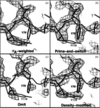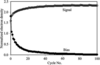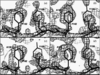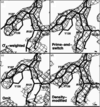## research papersBIOLOGICALCRYSTALLOGRAPHY
ISSN: 1399-0047
Volume 60| Part 12| December 2004| Pages 2144-2149

## Using prime-and-switch phasing to reduce model bias in molecular replacement

aBioscience Division, Mail Stop M888, Los Alamos National Laboratory, Los Alamos, NM 87545, USA
*Correspondence e-mail: terwilliger@lanl.gov

(Received 10 January 2004; accepted 9 August 2004)

Atomic models are commonly used to calculate phases in macromolecular crystallography. When combined with measured amplitudes, model-based phases yield electron-density maps with features of the correct structure but with a significant bias towards features of the model. The present contribution shows applications of the technique of prime-and-switch phasing to reduce this bias. An atomic model is used to generate phases that are close to the correct set but that may be biased. An unbiased source of phase information, an estimate of the probability that the electron-density map corresponds to a macromolecule, is then used to select a set of phases that are near the biased set, without further reference to the biased phases. The probability that the electron-density map corresponds to a macromolecule is based on agreement of the map with expectations such as a flat solvent region. Prime-and-switch phasing can be useful even for crystals with low solvent content and may reduce errors in interpretation of electron density in a wide range of applications of macromolecular crystallography, including molecular replacement, model building, ligand-binding and conformation-change studies, refinement and structure validation.

### 1. Introduction

Model bias is a very significant problem in macromolecular crystallography because it can lead to misinterpretation of electron-density maps, even when the maps are relatively accurate overall (Ramachandran & Srinivasan, 1961; Read, 1986; Bhat, 1988; Hodel et al., 1992; Adams et al., 1999; Kleywegt, 2000). It can occur in many stages of crystallographic analysis and is particularly important in molecular replacement (Rossmann, 1972), model building (Perrakis et al., 1999), ligand-binding and conformation-change studies and structure validation (Kleywegt, 2000). Methods for reducing model bias in electron-density map calculations have been developed (Ramachandran & Srinivasan, 1961; Read, 1986; Bhat, 1988; Hodel et al., 1992), but none are entirely satisfactory because each either removes only part of the effect of model bias or substantially increases the overall error in the maps.

Non-crystallographic symmetry, flat regions corresponding to disordered solvent and other features of electron-density maps have been used for some time as important sources of phase information in macromolecular crystallography (Rossmann, 1972; Bricogne, 1976; Wang, 1985; Xiang et al., 1993; Cowtan & Main, 1993; Szöke, 1993; Abrahams & Leslie, 1996; van der Plas & Millane, 2000; Béran & Szöke, 1995). We have recently developed a map-probability function that can be used to calculate phase probabilities based only on the presence of such features in the electron-density map (Terwilliger, 2001) and have described how it might be used to reduce model bias using the technique of `prime-and-switch' phasing. Here, we provide examples of the use of prime-and-switch phasing in molecular replacement and analyze the reduction in model bias that is obtained.

The map-probability function (Terwilliger, 2001) describes the plausibility of the electron-density map. If the electron density in the map is consistent with expectations (e.g. the solvent region is flat) then the probability that the electron-density map corresponds to a macromolecule is high and if the map is inconsistent with expectations the probability is low. The map-probability function is a simple way to put density-modification techniques on a sound statistical footing. It allows the calculation of phase probabilities from the relative probability that the electron-density map corresponds to a macromolecule for each possible value of a phase.

As described below, the phase probability for a reflection calculated with the map-probability function is unbiased by any prior estimates of the phase. At the same time, the more accurate the starting phases of all reflections, the more accurately the phase of each individual reflection can be determined. The combination of these properties makes a prime-and-switch phasing strategy for accurate and unbiased phase calculation possible.

### 2. Methods

Prime-and-switch phasing in molecular replacement is carried out in three steps. Firstly, crystallographic phases are calculated from an atomic model. It is anticipated that these phases are close to the correct set of phases, but that they are biased in a systematic way towards the coordinates of the atoms in the model. An initial electron-density map is calculated from these phases and measured amplitudes using σA-weighting (Read, 1986). Regions of the map containing solvent, macromolecule or other features are identified as in other density-modification procedures (Wang, 1985; Terwilliger, 2000) and plausible values of electron density in each such region are obtained from histograms of their values in this map. Next, the probability that the electron-density map corresponds to a macromolecule is estimated as described previously (Terwilliger, 2001), along with first and second derivatives of the probability with respect to each crystallographic phase. Finally, crystallographic phases are iteratively adjusted so as to maximize the overall probability that the electron-density map corresponds to a macromolecule. In this process, the original phase information is only used to calculate the starting electron-density map. This information is not included in the phase-improvement procedure as it is in other density-modification procedures. The number of iterations carried out is typically 50–100; smaller numbers of iterations generally lead to higher residual bias in the map (see §3), while extended iteration can result in poorer overall accuracy of the map.

### 3. Results

Fig. 1and Table 1illustrate the use of prime-and-switch phasing in one of its important potential applications. Molecular replacement (Rossmann, 1972) and associated techniques, such as difference Fourier analysis, are used for the solution of the majority of macromolecular structures. A key step in molecular replacement is calculation of structure factors using the molecular-replacement model. These phases and amplitudes are then used along with the measured amplitudes of structure factors to calculate an electron-density map that is to be used for building a new model. Any bias in this map towards the molecular-replacement model reduces the probability of building a correct model.

 Table 1Normalized signal and bias in electron-density maps
Structure/map type Refined coordinates(signal ± SEM) Incorrect coordinates(bias ± SEM) Mapcorrelation§
IF5A
N 1062 81
σA-weighted 1.46 ± 0.03 1.03 ± 0.10 0.48
Prime-and-switch 2.31 ± 0.04 0.01 ± 0.07 0.74
Density-modified 2.11 ± 0.04 0.89 ± 0.11 0.68
dhlA
N 2731 272
σA-weighted 1.59 ± 0.02 1.58 ± 0.04 0.48
Prime-and-switch 2.12 ± 0.03 0.55 ± 0.05 0.61
Density-modified 2.04 ± 0.02 1.89 ± 0.05 0.60
†The signal is calculated as the mean electron density at the coordinates of correctly placed atoms (as defined in the text), after subtraction of the overall mean of the map, normalized to the r.m.s. of the map. The bias is correspondingly calculated as the mean electron density at the coordinates of incorrectly placed atoms.
‡SEM indicates the standard error in the mean; N is the number of atoms in each category.
§The map correlation is the correlation of each map with an Fcalcexp(iφcalc) map based on the refined coordinates of the corresponding structure (IF5A or dhlA).Figure 1 Comparison of electron-density maps in molecular-replacement phasing for crystals with 60% solvent. All maps were calculated using the coordinates of M. jannaschii IF5A (PDB code 2eif ; Berman et al., 2000; Kim et al., 1998) and measured amplitudes from crystals of P. aerophilum IF5A (PDB code 1bkb ; Peat et al., 1998). Coordinates of M. jannaschii IF5A are in gray (including the labeled residue Tyr119) and those of P. aerophilum IF5A are in black (including the labeled residue Tyr79). (a) σA-weighted (Read, 1986) (2mFobs − DFcalc)exp(iφcalc) map. (b) Prime-and-switch map. (c) Omit map. (d) Statistical density-modification-based (Terwilliger, 2000) map. Contours are at 1.0σ in the omit map and 1.5σ in all others.

In Fig. 1and Table 1, the structure of initiation factor 5A (IF5A) from Methanococcus jannaschii (Kim et al., 1998) is used as a molecular-replacement model in phasing calculations using measured amplitudes of structure factors from 20 to 2.2 Å from crystals of IF5A from Pyrobaculum aerophilum (Peat et al., 1998). When superimposed, the two refined structures have an r.m.s. deviation of 1.7 Å overall (Gibrat et al., 1996) and the P. aerophilum crystals contain about 60% disordered solvent. Fig. 1(a) shows a σA-weighted (2mFobs − DFcalc)exp(iφcalc) map (Read, 1986) calculated from the model of M. jannaschii IF5A and using measured amplitudes from P. aerophilum IF5A crystals. Overlaid on the σA-weighted electron-density map are the coordinates of atoms in M. jannaschii IF5A used to initiate molecular-replacement phasing (gray) and atoms in the refined structure of P. aerophilum IF5A (black). The σA-weighted electron-density map closely matches the model (gray) from M. jannaschii that was used to calculate it and fails to match the refined structure (black) from P. aerophilum. The prime-and-switch phased electron-density map shown in Fig. 1(b) shows essentially no model bias towards the M. jannaschii IF5A model (gray) and very closely matches the refined structure (black) of P. aerophilum IF5A. Fig. 1(c) shows a σA-­weighted omit map calculated without using any atoms in the residues shown in Figs. 1(a) and 1(b). The omit map is very noisy and is not readily interpretable. Fig. 1(d) shows that solvent flattening using statistical density modification (Terwilliger, 2000) leads to a map that is approximately midway between the σA-weighted map and the prime-and-switch phased map, consistent with the inclusion of both model-based and map-based phase information with equal weighting.

Table 1analyzes the overall signal and bias present in the maps shown in Fig. 1. The signal was evaluated on the basis of the electron density at coordinates of atoms in the refined model of P. aerophilum IF5A. The bias was evaluated from the electron density at coordinates of atoms in the model from M. jannaschii IF5A that did not match any atom in the refined structure of P. aerophilum IF5A. These incorrectly placed atoms were selected to be those atoms in M. jannaschii IF5A that were in the electron-density range −0.25σ to 0.25σ in an Fcalcexp(iφcalc) map calculated from the refined structure of P. aerophium IF5A. Table 1shows that the prime-and-switch phased map in Fig. 1(b) had a greater signal (2.31σ) than the σA-weighted map shown in Fig. 1(a) (1.46σ) and much less bias (0.01σ compared with 1.03σ). The density-modified map in Fig. 1(d) had a signal of 2.11σ, nearly as high as that of the prime-and-switch phased map (2.31σ), but also a high bias (0.89σ), almost as large as that of the σA-weighted map (1.03σ).

Fig. 2shows that the iterative application of map-probability phasing is essential to remove the collective bias present in the model-based phases used to initiate prime-and-switch phasing. The overall signal and bias is shown for the 100 cycles of map-probability phasing used to obtain the map shown in Fig. 1(b). The signal improves slightly over the course of iterative map-probability phasing and the bias decreases from initial levels that are as high as the signal to nearly zero.Figure 2 Reduction of bias by iterative map-probability phasing. The signal and bias (as defined in Table 1) in prime-and-switch electron-density maps for P. aerophilum IF5A are primed with phases based on M. jannaschii IF5A calculated as in Fig. 1, as functions of the number of iterations of map-probability phasing.

The application of prime-and-switch phasing to a crystal with low solvent content is shown in Fig. 3. The structure of the dehalogenase linB from Sphingomonas paucimobilis (Marek et al., 2000) was used to initiate molecular-replacement phasing based on X-ray data from 20 to 1.5 Å from crystals of the Rhodococcus dehalogenase dhlA (Newman et al., 1999). When superimposed, the two structures have an r.m.s. deviation of 1.4 Å (Shindyalov & Bourne, 1998). The crystals of dhlA contain 30% solvent.Figure 3 Comparison of electron-density maps in molecular-replacement phasing for crystals with 30% solvent. The map is calculated using coordinates of linB from S. paucimobilis (PDB code 1cv2 ; Marek et al., 2000) and measured amplitudes from crystals of Rhodococcus dhlA (PDB code 1bn7 ; Newman et al., 1999). Coordinates of linB are in gray (including the labeled residues Ala135 and Tyr130) and those of dhlA are in black (including the labeled residues Phe142 and Cys139). Maps (a)–(d) are as in Fig. 1. Contours are at 1.0σ.

Fig. 3shows the σA-weighted map and the prime-and-switch map, each with the coordinates of the linB model superimposed in gray and those of the refined dhlA structure in black. The σA-weighted map shows substantial bias towards the model used to calculate it, while the prime-and-switch map shows much less model bias. Table 1shows the overall signal and bias in the maps shown in Fig. 3, estimated in the same fashion as for the maps in Fig. 1. The σA-weighted map has a bias of 1.58σ, about as large as the signal (1.59σ), while the prime-and-switch map has about a lower bias of 0.55σ and a signal (2.12σ) that is much stronger than that found in the σA-­weighted map (1.59σ). Unlike the case with 60% solvent, the prime-and-switch phasing procedure does not fully converge in this example; rather, the phases change rapidly during the first 25 cycles and then very gradually after that. Approaches for choosing the optimal number of iterations and additional detailed analyses of map-probability phasing have been discussed recently (Terwilliger, 2001); in this example, 50 cycles were carried out. After 100 cycles, the signal (defined as in Table 1) had decreased to from 2.1σ to 1.9σ and the bias from 0.55σ to 0.35σ; after 500 cycles, the signal in the map was reduced to 0.9σ and the bias to 0.14σ. In this example both the signal and the bias eventually decrease; the utility of the method lies in the observation that the signal decreases very slowly while the bias decreases rapidly. Consequently, an intermediate number of cycles can be carried out, leading to a map where the signal is still very strong and the bias is low.

The reduction of bias shown in Figs. 1and 3is principally at the sites of side chains that are different in the model and the correct structure. Fig. 4shows that this reduction of bias can also be substantial at the coordinates of main-chain atoms. The figure shows a section of the same map illustrated in Fig. 1, in this case in a region where the main chain is shifted slightly between the model of M. janaschii IF5A and the refined structure of P. aerophilum IF5A. As in Fig. 1, the σA-weighted electron-density map closely matches the model (gray) that was used to calculate it (compare the gray main chain of the model of M. janaschii IF5A at Gln125 with the black of the P. aerophilum IF5A at Arg131). In contrast, the prime-and-switch phased electron-density map (Fig. 4b) closely matches the refined structure (black) of P. aerophilum IF5A.Figure 4 Electron-density maps in the region where the main chain is offset. Sections from the same set of maps shown in Fig. 1are illustrated. Coordinates of M. jannaschii IF5A in gray (including the labeled residue Gln125) and of P. aerophilum IF5A in black (including the labeled residue Arg131).

### 4. Discussion

There are two premises behind prime-and-switch phasing as a tool for removing model bias. The first is that the phases calculated from an atomic model such as that which might be used in molecular replacement are fairly close to the correct ones and that the problem is not so much of phase accuracy but of phase bias. The second premise is that once crystallographic phases are known approximately then the plausibility of the electron-density map can, by itself, be used to adjust the phases in such a way that they stay close to the correct ones, while losing the memory of the model used to prime the process. These premises are supported by the substantial reduction in model bias that is found when the prime-and-switch approach is applied to realistic molecular-replacement cases.

One of the reasons why prime-and-switch phasing is able to remove model bias is that the map-probability estimate of the phase of a reflection is unbiased by prior estimates of this phase. This lack of direct bias occurs because of intrinsic cross-validation in the phase-calculation process (Terwilliger, 2000). Conceptually, a starting set of phases is used with measured amplitudes of structure factors to calculate an electron-density map using all reflections except one. Possible values of the phase of this reflection are sampled and for each phase a new electron-density map is constructed in which the contribution from this reflection is added to the map already calculated using all the others. The new map is examined to see how well it agrees with prior expectations about the map and the probability that the electron-density map corresponds to a macromolecule reflects the probability of this value of the phase. As this reflection is not used in calculating the starting electron-density map, the map-probability estimate of the phase of this reflection is not biased by prior estimates of this phase.

There are other types of bias that can affect map-probability phasing, however. If the set of phases used to initiate map-probability phasing has been adjusted as a whole in a way that leads to a flat solvent region, then map-probability phasing will tend to find these starting phases to be probable ones, because each leads to a flat solvent when combined with all the other starting phases. Map-probability phases can also be affected by an incorrect definition of the expectations about the map. As shown above, these types of bias can be reduced by iterative application of map-probability phasing.

Prime-and-switch phasing depends on having some starting phase information. In the examples shown in Figs. 1–4, the atomic model used to calculate phases differed from the refined model by an r.m.s. deviation of 1.4–1.7 Å. The more accurate the starting phase set, the more accurate the resulting map-probability phases, because map-probability phasing depends on interference between each structure factor and all others. For example, if there is considerable error in all phases then the solvent region will not be completely flat for any value of the phase of a particular reflection and it would be very difficult to specify which phase is most likely. Conversely, if the phases of all reflections but one were sufficiently accurate, then the requirement of a flat solvent in even a tiny region of the unit cell would allow an unambiguous determination of the phase of the remaining reflection.

Prime-and-switch phasing can reduce the effects of model bias, and methods to accomplish this are likely to become increasingly important as the number of known macromolecular structures and the use of molecular replacement and automated model building increase. As illustrated in Fig. 3, prime-and-switch phasing can be useful even for crystals with low solvent content. The approach may reduce errors in interpretation of electron density in a wide range of applications of macromolecular crystallography, including molecular replacement (Rossmann, 1972), model building and refinement (Perrakis et al., 1999), ligand-binding and conformation-change studies and structure validation (Kleywegt, 2000). A variation on prime-and-switch phasing is implemented in iterative model rebuilding with RESOLVE (Terwilliger, 2003). Prime-and-switch phasing may also be useful in general phase-recovery problems (Millane, 1990) in which an accurate but biased model is available to initiate phase recovery and insufficient support is present to recover phases in the absence of a model.

### Acknowledgements

The author is grateful for generous grant support from the US DOE and the National Institutes of Health and for helpful discussions with Joel Berendzen, Li-Wei Hung, Elaine Liong and Jean-Denis Pedelacq.

### ReferencesBIOLOGICALCRYSTALLOGRAPHY